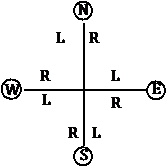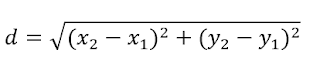# Some Important IQ Question with Solution and Reasons

## Example 1:

(a) 18739   (b) 18732   (c) 19700   (d) 20205

Solution: Clearly moving the clockwise we observe the following pattern.
1×1=1+1=2
2×2=4+2=6
6×3=18+3=21
21×4=84+4=88
88×5=440+5=445
445×6=2670+6=2676
So required number is 2676×7=18732+7= 18739

## Example: 2(a) 20   (b) 23   (c) 60   (d) 56
Solution: Clearly the digit 62 is exchange and will be 26 that’s why the answer is (b)

## Example: 3(a) 169   (b) 196   (c) 144   (d) 225
Solution: Clearly
2+3=5 & (5×5) =25
So 7+6=13 & (13×13)=169. Therefore, the answer is (a)

## Example: 4(a) 4   (b) 5   (c) 6   (d) 7
Solution: Clearly
10-8=2, 6-4=2 & 2+2=4
14-10=4, 8-7=1& 4+1=5, Therefore answer is (b)

## Example (1)

 A C F B D G C E ?

a) H   b) I   c) G   d) J
Solution: The differences between A and C is 2, C and F is 3 that’s why answer is H.

## Example (2)

 C E O D F X B J ?

a) S   b) T   c) W   d) L
Solution: Multiply first and second Alphabet then will be the third Alphabet C=3, E=5 Then 3×5=15. 15 is called ‘O’, Therefore B=2, J=10 Then 2×10=20. So, the answer is option b (T).

## Example (3)

 E J C K J I P T ?

a) J   b) O   c) L   d) K
Solution: 1st (E) + 2nd (K) =3rd (P), So C+I=L. Therefore, answer is option C.

## Example 1:

 6 18 5 15 3 12 6 24 4 16 5 20 8 24 7 ?

a) 21 b) 20 c) 9 d) 12
Solution:. In the first column 18/6=3, 3×5 =15
Therefore 24/8=3, 3×7=21. So, the answer is option a.

## Example 2:

 6 6 8 5 7 5 4 3 ? 120 126 320

a) 4 b) 12 c) 8 d) 16
Solution: In the first column 6×5×4=120
Let the missing number 8×5×? =320. Therefore, 320/40=8. So, the correct answer is option C.

## Example 3:

 4 3 2 1 9 5 2 5 3 21 115 ?
a) 38 b) 40 c) 30 d) 35
Solution: In the first column, (4×4)+(1×1)+(2×2)=16+1+4=21
In the third column, (2×2)+(5×5)+(3×3)= 4+25+9= 38
Therefore, the correct answer is option A.

## Direction and Distance

There are four major directions – North, South, East and West
North is the direction you are facing, and South is the direction at your back.
West is the direction in your left and East is the direction in your right.
Hints for salving direction problems.

1. For right and left.2. When the sun rises from east in the morning, the shadow of every objects is seen in the west until noon.
3. At the noon, there are no shadows.
4. After the noon, the shadow of every object is seen in the east.
5. Always refer North if the question doesn’t fix the direction.
6. If the question doesn’t fix the direction but mention distance at that time refer North.
7. If the question gives two objects, persons or letter than place the first object (any things) in the North and the second one in South.
8.If the question provides he distance between AB and BC, then you can calculate the distance AC by using following formula:h2=p2+b2
9. If the question asks to calculate total covered distance than, we can calculate by adding the distance from initial point to final point.
10. If the question provides grid reference (G.K) between two places, use the following formula.11. In the case of degree
Clockwise = R.H.S.Anti-clockwise L.H.S12. About turn means turn to 180°
13. Complete turn means turn to 360° or 0°

## Example Questions

1. From a initial point Sachin turns his right. He turns his left. In which direction is he facing now ?
(a) North   (b) North-East   (c) North-West   (d) South
Ans (a)

2. A walks 1 km. He turns right and walks 5 km. Again he turns to south and walks 4 km. At last he turns westwards and walks 9 km. In which direction is he from the initial point and how far is he from the starting point ?
(a) South-West 5 km.   (b) North 10 km.   (c) 7 km. South   (d) South Eat 5 km.
Ans (a)